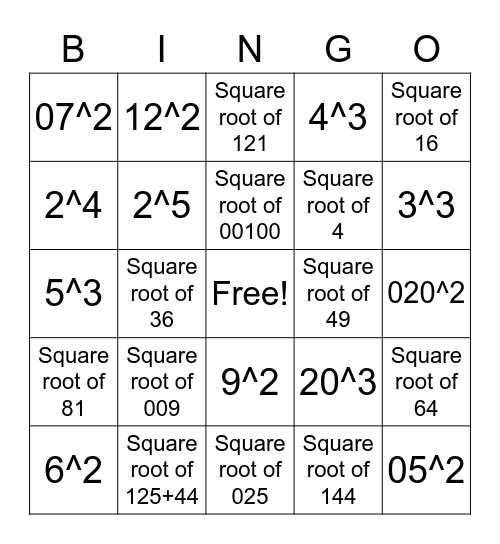# Square RootThis bingo card has a free space and 24 words: Square root of 009, Square root of 125+44, Square root of 00100, 6^2, Square root of 16, 07^2, 2^4, 12^2, Square root of 49, 9^2, Square root of 4, 5^3, 20^3, Square root of 64, 2^5, Square root of 144, 020^2, Square root of 121, 3^3, Square root of 36, 05^2, Square root of 81, 4^3 and Square root of 025.

## Play Online